Next: Boundary conditions Up: Time accuracy Previous: Grid   Contents

### Initial conditions

The initial velocity field is quiescent, the initial free surface height is 0, and the initial density distribution is defined in the function ReturnSalinity in initialization.c as

  return -.03*tanh(2.0*2.6467/20*(z+50-cos(PI*x/100)));

Because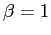in suntans.dat (the parameter beta), this is also the initial density distribution, since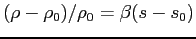More generally, then, this density distribution is given by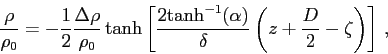where
•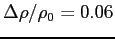: Density difference between layers.
•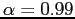: Parameter that defines interface extent.
•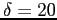m: Interface thickness.
•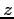: Vertical coordinate
•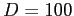m: Depth
•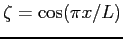: Interface profile, where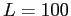m is the domain length.
and is shown in Figure 7.
 1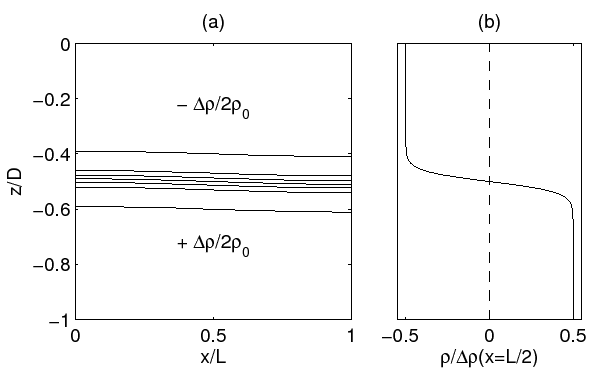2014-08-06